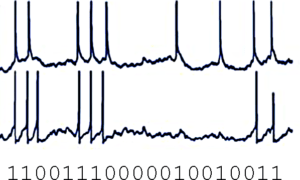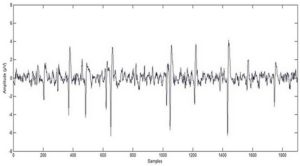# A new way to computeI read an article the other day on using using random pulses rather than digital numbers to compute with, see Computing with random pulses promises to simplify circuitry and save power, in IEEE Spectrum. Essentially they encode a number as a probability in a random string of bits and then use simple logic to compute with. This approach was invented in the early days of digital logic and was called stochastic computing.

# Stochastic numbers?

It’s pretty easy to understand how such logic can work for fractions. For example to represent 1/4, you would construct a bit stream that had one out of every four bits, on average, as a 1 and the rest 0’s. This could easily be a random string of bits which have an average of 1 out of every 4 bits as a one.

A nice result of such a numerical representation is that it easily results in more precision as you increase the length of the bit stream. The paper calls this progressive precision.

Progressive precision helps stochastic computing be more fault tolerant than standard digital logic. That is, if the string has one bit changed it’s not going to make that much of a difference from the original string and computing with an erroneous number like this will probably result in similar results to the correct number.  To have anything like this in digital computation requires parity bits, ECC, CRC and other error correction mechanisms and the logic required to implement these is extensive.

# Stochastic computing

Another advantage of stochastic computation and using a probability  rather than binary (or decimal) digital representation, is that most arithmetic functions are much simpler to implement.

They discuss two examples in the original paper:

Multiplication – Multiplying two probabilistic bit streams together is as simple as ANDing the two strings.

Addition – Adding two probabilistic bit strings together just requires a multiplexer, but you end up with a bit string that is the sum of the two divided by two.

I see a couple of problems with stochastic computing:,

• How do you represent  an irrational number, such as the square root of 2;
• How do you represent integers or for that matter any value greater than 1.0 in a probabilistic bit stream; and
• How do you represent negative values in a bit stream.

I suppose irrational numbers could be represented by taking a near-by, close approximation of the irrational number. For instance, using 1.4 for the square root of two, or 1.41, or 1.414, …. And this way you could get whatever (progressive) precision that was needed.

As for integers greater than 1.0, perhaps they could use a floating point representation, with two defined bit strings, one representing the mantissa (fractional part) and the other an exponent. We would assume that the exponent rather than being a probability from 0..1.0, would be inverted and represent 1.0…∞.

Negative numbers are a different problem. One way to supply negative numbers is to use something akin to complemetary representation. For example, rather than the probabilistic bit stream representing 0.0 to 1.0 have it represent -0.5 to 0.5. Then progressive precision would work for negative numbers as well a positive numbers.

One major downside to stochastic numbers and computation is that high precision arithmetic is very difficult to achieve.  To perform 32 bit precision arithmetic would require a bit streams that were  2³² bits long. 64 bit precision would require streams that were  2**64th bits long.

# Good uses for stochastic computing

One advantage of simplified logic used in stochastic computing is it needs a lot less power to compute. One example in the paper they use for stochastic computers is as a retinal sensor for in the body visual augmentation. They developed a neural net that did edge detection that used a stochastic front end to simplify the logic and cut down on power requirements.

Other areas where stochastic computing might help is for IoT applications. There’s been a lot of interest in IoT sensors being embedded in streets, parking lots, buildings, bridges, trucks, cars etc. Most have a need to perform a modest amount of edge computing and then send information up to the cloud or some edge consolidator intermediateMany of these embedded devices lack access to power, so they will need to make do with whatever they can find.  One approach is to siphon power from ambient radio (see this  Electricity harvesting… article), temperature differences (see this MIT … power from daily temperature swings article), footsteps (see Pavegen) or other mechanisms.

The other use for stochastic computing is to mimic the brain. It appears that the brain encodes information in pulses of electric potential. Computation in the brain happens across exhibitory and inhibitory circuits that all seem to interact together.  Stochastic computing might be an effective way, low power way to simulate the brain at a much finer granularity than what’s available today using standard digital computation.~~~~

Not sure it’s all there yet, but there’s definitely some advantages to stochastic computing. I could see it being especially useful for in body sensors and many IoT devices.